﻿ Solution of Nonlinear Equations in Science through Lagrange’s Inversion Theorem

### Solution of Nonlinear Equations in Science through Lagrange’s Inversion Theorem

Pushpa N. Rathie, Prabhata K. Swamee, Luan Carlos de S. M. OzelimOPEN ACCESSPEER-REVIEWED

## Solution of Nonlinear Equations in Science through Lagrange’s Inversion Theorem

Pushpa N. Rathie1,, Prabhata K. Swamee2, Luan Carlos de S. M. Ozelim3

1Department of Statistics, University of Brasilia, Brasilia, Brazil

2Department of Civil Engineering, ITM University, Gurgaon, India

3Department of Civil and Environmental Engineering, University of Brasilia, Brasilia, Brazil

### Abstract

Nonlinear problems arise in most of the scientific fields. In general, such behavior is represented by a nonlinear equation, whose solution is sought. Analytical and numerical methods have been applied to the solution of this class of equations, notwithstanding, in cases where highly nonlinear phenomena are analyzed, the number of iterations and computational effort necessary to achieve the minimum required accuracy is very high. Lagrange´s Inversion Theorem (LIT) has been applied to solve this kind of problems analytically, giving the solution as an infinite power series. This way, the accuracy can be as high as necessary by taking more terms from the series solution, which is easily computationally implemented. Also, in some cases it is possible to relate the series obtained to the expansion of special and elementary functions, which enables one to exactly solve the desired equation. In the present review paper, a total of eleven applications have been discussed in order to show the role of LIT in various areas of nonlinear sciences.

• Rathie, Pushpa N., Prabhata K. Swamee, and Luan Carlos de S. M. Ozelim. "Solution of Nonlinear Equations in Science through Lagrange’s Inversion Theorem." Applied Mathematics and Physics 1.4 (2013): 120-125.
• Rathie, P. N. , Swamee, P. K. , & Ozelim, L. C. D. S. M. (2013). Solution of Nonlinear Equations in Science through Lagrange’s Inversion Theorem. Applied Mathematics and Physics, 1(4), 120-125.
• Rathie, Pushpa N., Prabhata K. Swamee, and Luan Carlos de S. M. Ozelim. "Solution of Nonlinear Equations in Science through Lagrange’s Inversion Theorem." Applied Mathematics and Physics 1, no. 4 (2013): 120-125.

 Import into BibTeX Import into EndNote Import into RefMan Import into RefWorks

### 1. Introduction

Modeling natural phenomena is one of the greatest challenges in every branch of Science. At first, when very little is known about the subject of study, linear approximations and descriptions tend to be the first approach made by scientists. On the other hand, when the phenomena of interest are further investigated, a few peculiarities which, at first, did not seem to be important have to be taken into account for building better models.

In general, when these particularities are considered, nonlinear equations arise. In the process of applying the new relations to the description of phenomena, the success intrinsically relies on the solution of the former. In order to address this issue, analytical and numerical methods are considered.

Since the advent of modern computer sciences, the usage of numerical methods has tremendously increased. This comes from the fact that computers became powerful tools of multiprocessing procedures and routines. On the other hand, as widely known, modeling systems do not depend on the solution of a single equation, but on a great number of them. In special, while considering nonlinear equations, the number of iterations necessary to obtain a desired accuracy is very high. This way, even if the numerical solution of a single equation by standard trial and error methods is worth the computational effort, the solution of large sets surely is not.

Analytical approaches, on the other hand, tend to be applicable only to special cases, in which it is possible to explicit the variable of interest by means of arithmetic manipulation. In order to address more complicated problems, an analytical exact method which does not depend on arithmetical manipulation is the Lagrange´s Inversion Theorem (LIT), which gives the exact analytical solution of a nonlinear equation by means of an infinite series. The theorem can be readily stated as follows : Let y be defined as the following function of constant, function φ, and a parameter δ: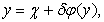(1)

then any function ζ(y) is expressed as the following power series in δ:(2)

It can be noticed that the right hand side of Eq.(2) contains y through δ defined in Eq.(1).

It is evident that the convergence issues concerning the series in Eq.(2) have to be taken into account for consistency of the solution. Besides, it is worth noticing that the right hand side of Eq.(2) does not depend on y, this way if one takes ζ(y) = y, the once implicit function y is now explicit.

In the presented paper, the usage of both Eqs.(1) and (2) is shown by discussing eleven applications of them in Civil Engineering, Mathematics, Statistics, Graph Theory and Chemical Engineering.

### 2. Examples of Application in Science

In this section a brief review of where LIT has been applied is shown. In order to better structure the paper, the applications are grouped following the area they are related to.

2.1. Civil Engineering

In the present subsection, applications in Civil engineering are shown. Since this paper is a review paper, the full theory behind each application is suppressed.

Regarding the alternate and sequent depths applications, one may refer to, for example, ,  and .

Regarding the three-parameter infiltration equation application, one may refer to  and .

2.1.1. Alternate Depths in a Triangular Shaped Channel

In order to obtain the alternate depths for a triangular shaped channel, the following equation has to be taken into account :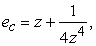(3)

in which, ec is a reduced energy parameter and z, a reduced depth parameter. Since Eq.(3) has two roots of interest (z1 and z2), one has to invert it accordingly, this way, in order to get the first root, Eq.(3) can be rearranged as:(4)

in which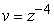. By means of LIT with ζ(v) = v-1/4, the value of z1 is given as: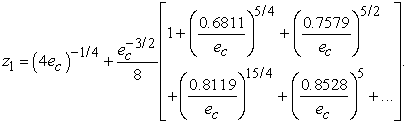(5)

On the other hand, in order to get the value of z2, Eq. (3) may be rearranged as: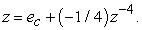(6)

This way, by means of LIT with ζ(z) = z, the value of z2 is: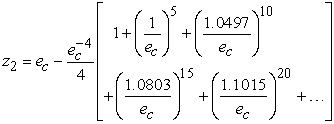(7)

Alternate Depths are a key parameter in the study of open channel flows.

2.1.2. Alternate Depths in a Parabolic Shaped Channel

In order to obtain the alternate depths for a parabolic shaped channel, the following equation has to be taken into account :(8)

in which, ec is a reduced energy parameter and z, a reduced depth parameter. As in the last subsection, Eq.(8) has two roots of interest (z1 and z2) thus one has to invert it accordingly. In order to get the first root, Eq.(8) can be rearranged as: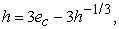(9)

in which. By means of LIT with ζ(h) = h-1/3, the value of z1 is given as: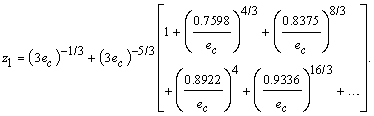(10)

On the other hand, in order to get the value of z2, Eq. (8) may be rearranged as: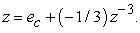(11)

This way, by means of Eq. (11) and LIT with ζ(z) = z, the value of z2 is:(12)

2.1.3. Sequent Depths in a Triangular Shaped Channel

In order to obtain the sequent depths for a triangular shaped channel, the following equation has to be taken into account :(13)

in which, mc is a reduced momentum parameter and z, a reduced depth parameter. There are two zeros of Eq. (13) which are of interest (z1 and z2) thus one has to invert it accordingly. In order to get the first root, Eq.(13) can be rearranged as: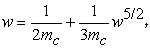(14)

in which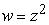. By means of LIT with ζ(w) = w1/2, the value of z1 is given as:(15)

On the other hand, in order to get the value of z2, Eq. (13) may be rearranged as:(16)

in which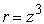. This way, by means of Eq. (16) and LIT with ζ(z) = r1/3, the value of z2 is: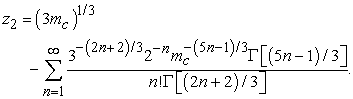(17)

Sequent Depths, as the Alternate Depths, are key parameters in the study of open channel flow.

2.1.4. Sequent Depths in a Parabolic Shaped Channel

In order to obtain the sequent depths for a parabolic shaped channel, the following equation has to be taken into account :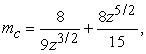(18)

in which, mc is a reduced momentum parameter and z, a reduced depth parameter. There are two zeros of Eq. (13) which are of interest (z1 and z2) thus one has to invert it accordingly. In order to get the first root, Eq.(18) can be rearranged as: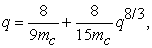(19)

in which. By means of LIT with ζ(q) = q2/3, the value of z1 is given as: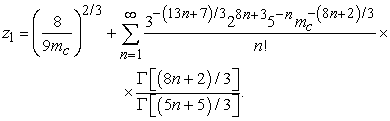(20)

On the other hand, in order to get the value of z2, Eq. (18) may be rearranged as: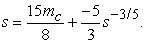(21)

in which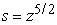. This way, by means of Eq. (16) and LIT with ζ(s) = s2/5, the value of z2 is: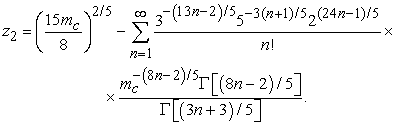(22)

2.1.5. Three-Parameter Infiltration Equation

In a recent paper, Rathie et al.  studied the three-parameter infiltration equation, stated as: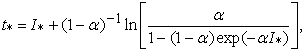(23)

in which I* is the nondimensional cumulative infiltration and t*, the nondimensional time. Also, α is a transition parameter which pertains the interval [0,1]. An approximate solution has been presented in .

In order to provide the nondimensional cumulative infiltration explicitely, Eq. (23) can be rearranged as: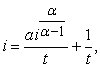(24)

in which i = exp((1- α)I*); t = αexp(t*(α – 1)); and a = α – 1. By means of Lagrange´s Inversion Theorem, with ζ(i) = i1/(1-α), the value of interest is given as: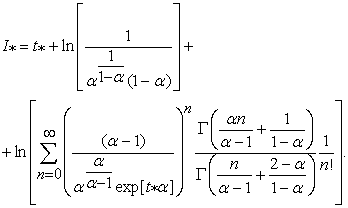(25)

Computational programs tend to misinterpret Eq.(25) as the gamma function arguments in the latter became negative integers. It is worth noticing that even if the arguments of individual gamma function in Eq. (25) became problematic, the ratio of the gamma function presented in the reffered equation does not. In order to provide a better way of implementing Eq.(25), let one define the Pochhammer symbol as:(26)

This way, by means of Eq. (26) and the multiplication formula for the gamma function, Eq.(25) is easily converted to: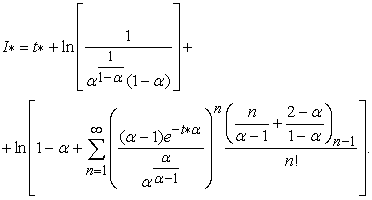(27)

More applications of LIT in Civil Engineering have been discussed in .

2.2. Algebraic Equations

In the present subsection, the applications related to obtaining solutions to algebraic equations are discussed. One may refer to ,  and .

2.2.1. Solution to Real Degree Equations

Recently, Rathie and Ozelim  derived the general solution to two classes of real degree equations. The solutions were obtained by means of LIT and subsequently have been converted to H-functions in order to provide a closed-form representation. Since the aim of the present paper is to review the applications of LIT, only the latter shall be discussed. This way, consider the general real degree equation of the following type: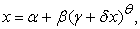(28)

in which α, β, γ and δ are arbitrary complex numbers and θ is a real number. By means of LIT, with ζ(x) = x, Eq. (28) can be solved for x as:(29)

On the other hand, consider the real degree equation of following type:(30)

in which β* and γ* are arbitrary complex numbers and α* and δ* are real numbers. It can be seen, by comparing Eq.(30) to Eq.(28) that, in general, one is not a special case of the other. Only if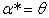,,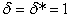,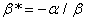and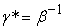they become explicitly correlated. Consider that Eq.(30) can be rewritten as:(31)

in which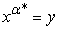.By means of LIT, with ζ(y) = yφ/α* and assuming sign(φ) = sign(α*), where φ is an arbitrary constant, Eq. (31) provides: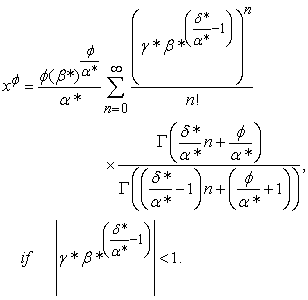(32)
2.3. Statistics

In the present subsection, applications in statistics are discussed. In order to obtain further information about the generalized logistic distribution, one may refer to ,  and .

2.3.1. New Skew Generalized Logistic Distribution

Let the probability density function of the Skew generalized Logistic Distribution be defined as follows :(33)

where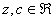and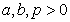. For the special case in which c = -1, Eq. (33) provides: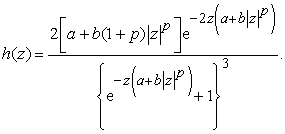(34)

The cumulative distribution function is obtained by means of Eq. (34) as: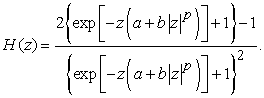(35)

In order to get z as a function o H, Eq. (35) shall be rearranged as:(36)

Finally, by applying LIT to Eq. (36), z is explicitly given as: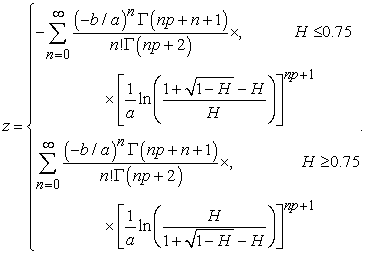(37)

2.3.2. Generalized Loglogistic Distribution

Rathie et al.  discussed the cummulative distribution function of the Generalized Loglogistic distribution, defined as:(38)

where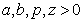.

In order to get z as a function of F, Eq. (38) may be rewritten as: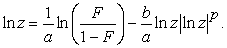(39)

By applying LIT to Eq. (39), the following is obtained:(40)
2.4. Graph Theory

In the present subsection, applications in Graph theory are discussed. For further information regarding the theory behind the enumeration of certain maps, one may refer to ,  and .

2.4.1. Enumeration of Almost Cubic Maps

While studying the enumeration of almost cubic maps, the following equation has been obtained by Mathai and Rathie :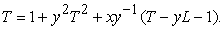(41)

In order to solve Eq. (41) for T, the following rearrangement has to be taken into account: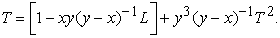(42)

By applying LIT to Eq. (42), T is given as:(43)

It is worth noticing that Eq.(43) can be further simplified by means of the binomial expansion. Some applications of LIT in Graph Theory have been discussed by Rathie .

2.5. Chemical Engineering

In the present subsection, applications in chemical engineering are discussed. One may refer to  and  for further information about the equations solved.

2.5.1. Friction Factor Problems Involving Laminar Flow of Bingham Plastic Fluids

In a recent paper, Swamee et al.  analyzed the following implicit equation related to friction factor problems involving laminar flow of Bingham plastic fluids: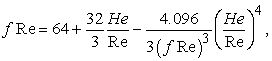(44)

in which f is the parameter of interest and Re and He are constants. By means of LIT, by taking ζ(f Re) = (f Re), Eq. (44) is solved as: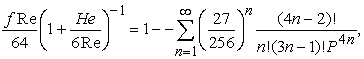(45)

where P = 1 + 6Re/He. One may notice that the series in Eq. (45) is fast converging for cases in which P (or He/Re is small). This way, one has to find another series which fastly converges when He/Re is large. Thus, consider the following rearrangement of Eq. (44):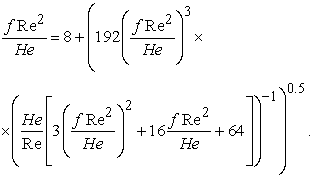(46)

Equation (46) can be readily inverted by means of LIT with ζ (f Re2/He) = f Re2/He as:(47)

This way, f is given explicitly. In order to define the applicability of both Eqs. (45) and (47), after an error analysis, Swamee et al.  showed that the former is valid for He/Re ≤ 30 and the latter, otherwise.

2.5.2. Diameter Problems Involving Laminar Flow of Bingham Plastic Fluids

Swamee et al.  also studied the diameter problems related to the laminar flow of Bingham plastic fluids by means of the following equation: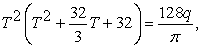(48)

in which T = D* - 4, D* is the parameter of interest and q is a constant. For small q, Eq. (48) can be inverted by means of LIT if one takes y = T2; ζ(y) = y-1/2; χ = π/4q; δ = π/128q and φ(y) = y-1/2(y-1/2 +32/3). This way, the solution process yields: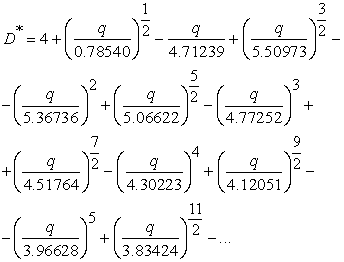(49)

On the other hand, for large values of q, by using LIT with y = T4; ζ(y) = y-1/4; χ = π/128q; δ = π/4q and φ(y) = y1/4/3 + y1/2; the value of D* is given as: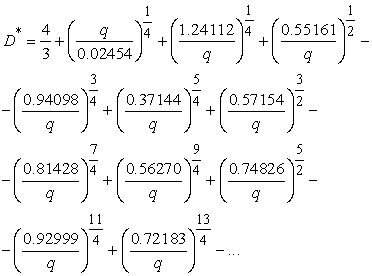(50)

This way, the series above give the diameter parameter D* explicitly. Swamee et al.  showed that, in order to diminish the error related to the applicability of both Eqs. (49) and (50), the former is valid for q ≤ 1.05 and the latter, otherwise.

### 3. Conclusions

Nonlinear problems are the core of most of Science’s branches. This way, analytical tools to provide exact solutions to nonlinear equations play a major role in each of these fields. Lagrange´s Inversion Theorem (LIT) shows up as a good alternative in the solution process. A total of eleven applications in four different fields have been discussed, and the importance of the solutions provided by LIT is widely recognized.

### Conflict of Interest

The authors declare that there is no conflict of interests regarding the publication of this article.

### References

  Bakhmeteff, B. A. Hydraulics of open channels, McGraw-Hill, New York, N.Y., 1932.In article  Chow, V. T. Open channel hydraulics, McGraw-Hill, Inc., New York, N.Y., 1959.In article PubMed  Cockle, J. Sketch of a theory of transcendental roots, London Edinburgh Dublin Philos. Mag. J. Sci. 20, pp.145-148, 1860.In article  Darby R,. Chemical Engineering Fluid Mechanics., Marcel Dekker, New York City, New York, pp 164-169, 1996.In article  Darby, R., Melson, J. How to predict the friction factor for flow of Bingham plastics. Chemical Engineering, 28: pp. 59-61, 1981.In article  Glasser, M. L. Hypergeometric functions and the trinomial equation, J. Comput. Appl. Math. 118, pp.169-173, 2000.In article CrossRef  Henderson, F. M. Open channel flow, The MacMillan Co., New York, N.Y., 1966.In article  King, R. B. Beyond the Quartic Equation, Birkhäuser, Boston, 1996.In article  Mathai, A. M.; Rathie, P. N., Enumeration of Almost Cubic Maps, Journal of Combinatorial Theory, Series B, Vol. 13 (1), p. 83-90, 1972.In article CrossRef  Mullin, R. C. On the average number of trees in certain maps. Canad. J. Math., 18, pp. 33-41, 1966.In article CrossRef  Parlange, J. Y., Barry, D. A., Haverkamp, R. Explicit infiltration equations and the Lambert W-function, Adv. Water Resour., 25 (8-12), pp. 1119-1124, 2002.In article  Parlange, J. Y., Lisle, I., Braddock, R. D., Smith, R. E. The three parameter infiltration equation, Soil Sci., 133 (6), pp. 337-341, 1982.In article CrossRef  Rathie, P. N. Normal distribution, Univariate, International Encyclopedia of Statistical Sciences, Springer Verlag, Part 14, pp. 1012-1013, 2011.In article  Rathie, P. N., On Some Enumeration Problems in Graph Theory, Annals of Discrete Mathematics, vol. 6, p. 281-288, 1980.In article CrossRef  Rathie, P. N., Swamee, P. K. On a new invertible generalized logistic distribution approximation to normal distribution, Technical Research Report in Statistics, 07/2006, Dept. of Statistics, Univ. of Brasilia, Brasilia, Brazil. 2006.In article  Rathie, P. N.; Coutinho, M. A new Skew Generalized Logistic distribution and Approximation to Skew Normal Distribution. Aligarh Journal of Statistics, v.31, p.1-12, 2011.In article  Rathie, P. N.; Ozelim, L. C. S. M. . General Solutions to Certain Real Degree Equations and their Applications. Advanced Studies in Contemporary Mathematics (Kyungshang), v. 22, p. 325-341, 2012.In article  Rathie, P. N.; Swamee, P. K.; Cavalcante, A. L. B.; Ozelim, L. C. S. M., Lagrange’s Inversion Theorem and Infiltration. World Academy of Science, Engineering and Technology, v. 67, p. 473-478, 2012.In article  Rathie, P. N.; Swamee, P. K.; Coutinho, M., Generalized Loglogistic Distribution and Its Applications. In: The 5th International Conference MSAST 2011 of IMBIC, 2011, Kolkata. Proceedings of The 5th International Conference MSAST 2011 of IMBIC, p. 88-99, 2011.In article  Rathie, P. N.; Swamee, P. K.; Ozelim, L. C. S. M.. Closed-form exact solutions in hydraulics by means of the H-Function. In: The 9th Annual Conference of the Society for Special Function and Their Application (SSFA), 2010, Gwalior. Proceedings of the 9th Annual Conference of the Society for Special Function and Their Application (SSFA), v. 9. p. 3-14, 2010.In article  Swamee, P. K., Rathie, P. N., Invertible Alternatives to Normal and Lognormal Distributions, Journal of Hydrologic Engineering, 12(2), pp. 218-221, 2007.In article CrossRef  Swamee, P. K.; Rathie, P. N.; Aggarwal, N., Exact solution of friction factor and diameter problems involving laminar flow of Bingham plastic fluids. Journal of Petroleum and Gas Exploration Research. v. 2(2), p. 27-32, 2012.In article  Swamee, P. K.; Rathie, P. N.; Ozelim, L. C. S. M., Analytical Solutions for Alternate Depths. ISH Journal of Hydraulic Engineering, v. 17, p. 34-42, 2011.In article CrossRef  Swamee, P. K.; Rathie, P. N.; Ozelim, L. C. S. M., Application of Analytical and Numerical Methods to the Sequent Depths Problem in Civil Engineering. Australian Journal of Basic and Applied Sciences, v. 5, p. 38-47, 2011.In article  Tutte, W. T. A census of planar maps. Canad. J. Math., 15, pp. 249-271. 1963.In article CrossRef  Tutte, W. T. On the enumeration of planar maps. Bull. Amer. Math. Soc., 74, pp. 64-74. 1968.In article CrossRef  Whittaker E. T., Watson G. N., A Course of Modern Analysis. 4th Edition, Cambridge Univ. Press, Cambridge, U.K., pg. 133, 1990.In article